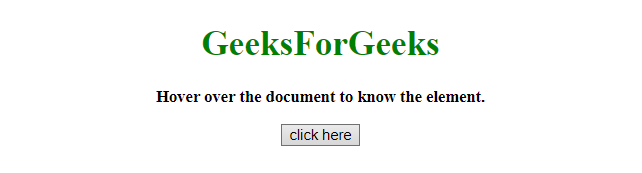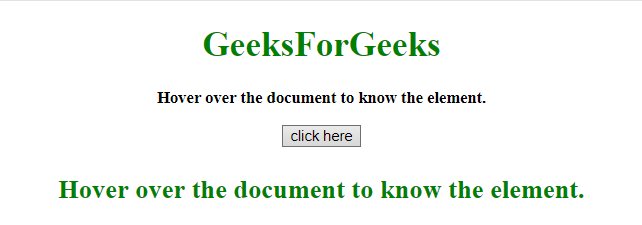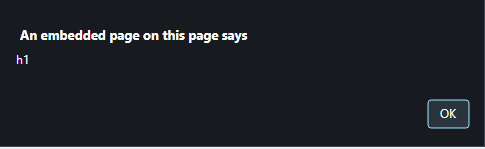GeeksforGeeks App
Open AppBrowser
Continue

# How to determine which element the mouse pointer move over using JavaScript ?

Given an HTML document and the task is to get the element where mouse pointer moves over. There are two approaches to solve this problem which are discussed below:

Approach 1:

• Get the x and y coordinates value by using .clientX and .clientY property.
• Use document.elementFromPoint(x, y) method to get the element content on that position when mouse pointer moves over.

Example 1: This example implements the above approach.

 ` ``<``html``> `` ` `<``head``> ``    ``<``title``> ``        ``How to determine which element the mouse``        ``pointer move over using JavaScript ?``    `` `` `` ` `<``body` `style` `= ``"text-align:center;"``> ``     ` `    ``<``h1` `style` `= ``"color:green;"` `onmouseover` `= ``"GFG_Fun()"``> ``        ``GeeksForGeeks ``    `` ``     ` `    ``<``p` `id` `= ``"GFG_UP"` `onmouseover` `= ``"GFG_Fun()"``            ``style` `= ``"font-size: 15px; font-weight: bold;"``> ``    ````     ` `    ``<``button` `onmouseover` `= ``"GFG_Fun()"``> ``        ``click here ``    `` ``     ` `    ``<``p` `id` `= ``"GFG_DOWN"` `style` `= ``        ``"font-size: 24px; font-weight: bold; color: green;"``> ``    ````     ` `    ``<``script``> ``        ``var up = document.getElementById('GFG_UP'); ``        ``var down = document.getElementById('GFG_DOWN'); ``        ``up.innerHTML = "Hover over the document to know the element."; ``         ` `        ``function GFG_Fun() { ``            ``var x = event.clientX;``            ``var y = event.clientY;``            ``el = document.elementFromPoint(x, y);``            ``down.innerHTML = el.innerHTML;``        ``} ``    `` ```` ` ``

Output:

• Before Hover on the button:• After Hover on the button:Approach 2:

• Attach the event ‘onmouseover’ to the element.
• Call the alert function with the id of that element, each time when event occurs.

Example 2: This example using the approach discussed above.

 ` ``<``html``> `` ` `<``head``> ``    ``<``title``> ``        ``How to determine which element the mouse``        ``pointer move over using JavaScript ?``    `` `` `` ` `<``body` `style` `= ``"text-align:center;"``> ``     ` `    ``<``h1` `id` `= ``"h1"` `style` `= ``"color:green;"``            ``onmouseover` `= ``"alert(this.id)"``> ``        ``GeeksForGeeks ``    `` ``     ` `    ``<``p` `id` `= ``"p"` `onmouseover` `= ``"alert(this.id)"``            ``style` `= ``"font-size: 15px; font-weight: bold;"``> ``        ``Hover over the document to know the element.``    ````     ` `    ``<``button` `id` `= ``"button"` `onmouseover` `= ``"alert(this.id)"``> ``        ``click here ``    `` `` `` ` ``

Output:

• Before Hover on the button:• After Hover on the button:My Personal Notes arrow_drop_up
Related Tutorials